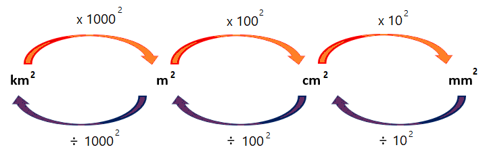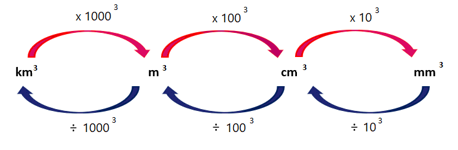Home Practice
For learners and parents For teachers and schools
Textbooks
Full catalogue
Pricing SupportLog in

We think you are located in United States. Is this correct?

# Converting between different units

## 17.6 Converting between different units

Surface area is measured in square units. Surface area can be expressed in square millimetres ($$\text{mm}^2$$), square centimetres ($$\text{cm}^2$$), square metres ($$\text{m}^2$$) and also square kilometres ($$\text{km}^2$$).

The diagram below shows how to convert between the different units of area.## Worked Example 17.10: Converting between units of area

Convert $$157 \text{ mm}^2$$ to square centimetres.

### Divide the area by $$100$$.

To convert from square millimetres to square centimetres, we divide the area by $$100$$:

\begin{align} 157 \text{ mm}^2 &= 157 \div 100 \\ &= \text{1,57} \text{ cm}^2 \end{align}

## Worked Example 17.11: Converting between units of area

Convert $$\text{3,08} \text{ m}^2$$ to square centimetres.

### Multiply the area by $$100^{2}$$.

To convert from square metres to square centimetres, we multiply the area by $$100^2$$:

\begin{align} \text{3,08} \text{ m}^2 &= \text{3,08} \times \text{10 000} \\ &= \text{30 800} \text{ cm}^2 \end{align}
Exercise 17.1

Convert each area to square centimetres.

1. $$\text{2,06} \text{ m}^2$$
2. $$\text{7 423} \text{ mm}^2$$
3. $$\text{0,015} \text{ m}^2$$
4. $$\text{842,5} \text{ mm}^2$$
5. $$\text{0,416} \text{ m}^2$$
1. $$\text{20 600} \text{ cm}^2$$
2. $$\text{74,23} \text{ cm}^2$$
3. $$\text{150} \text{ cm}^2$$
4. $$\text{8,425} \text{ cm}^2$$
5. $$\text{4 160} \text{ cm}^2$$

Convert each area to square millimetres.

1. $$\text{3,4} \text{ cm}^2$$
2. $$\text{0,009} \text{ m}^2$$
3. $$\text{0,62} \text{ cm}^2$$
4. $$\text{0,00045} \text{ m}^2$$
5. $$\text{72,6} \text{ cm}^2$$
1. $$\text{340} \text{ mm}^2$$
2. $$\text{9 000} \text{ mm}^2$$
3. $$\text{62} \text{ mm}^2$$
4. $$\text{4 500} \text{ mm}^2$$
5. $$\text{7 260} \text{ mm}^2$$

Convert each area to square metres.

1. $$\text{1 753} \text{ cm}^2$$
2. $$\text{85 000} \text{ mm}^2$$
3. $$\text{55 010} \text{ cm}^2$$
4. $$\text{2,04} \text{ km}^2$$
5. $$\text{360} \text{ cm}^2$$
1. $$\text{0,1753} \text{ m}^2$$
2. $$\text{0,085} \text{ m}^2$$
3. $$\text{5,501} \text{ m}^2$$
4. $$\text{2 040 000} \text{ m}^2$$
5. $$\text{0,036} \text{ m}^2$$

Volume is measured in cubic units. The volume of an object can be expressed in cubic millimetres ($$\text{mm}^3$$), cubic centimetres ($$\text{cm}^3$$), cubic metres ($$\text{m}^3$$) and also cubic kilometres ($$\text{km}^3$$).

The diagram below shows how to convert between the different units of volume.## Worked Example 17.12: Converting between units of volume

Convert $$\text{8 259} \text{ mm}^3$$ to cubic centimetres.

### Divide the volume by $$10^{3}$$.

To convert from cubic millimetres to cubic centimetres, we divide the volume by $$10^3 = 1\ 000$$:

\begin{align} \text{8 259} \text{ mm}^3 &= \text{8 259} \div \text{1 000} \\ &= \text{8,259} \text{ cm}^3 \end{align}

## Worked Example 17.13: Converting between units of volume

Convert $$\text{3,08} \text{ m}^3$$ to cubic centimetres.

### Multiply the volume by $$100^{3}$$.

To convert from cubic metres to cubic centimetres, we multiply the volume by $$100^3 = 1\ 000\ 000$$:

\begin{align} \text{3,08} \text{ m}^3 &= \text{3,08} \times \text{1 000 000} \\ &= \text{3 080 000} \text{ cm}^3 \end{align}

Capacity can be expressed in millilitres ($$\text{ml}$$), litres ($$\text{l)}$$ and kilolitres ($$\text{kl}$$). Capacity can also be measured in cubic centimetres ($$\text{cm}^3$$) and cubic metres ($$\text{m}^3$$).

• $$1 \text{ cm}^3 = 1 \text{ ml}$$
• $$1\ 000 \text{ cm}^3 = 1 \text{ litre}$$

## Worked Example 17.14: Converting between units of volume and capacity

Convert $$560 \text{ cm}^3$$ to litres.

### Divide the volume by $$1\ 000$$.

To convert from cubic centimetres to litres, we divide the volume by $$1\ 000$$:

$1 \text{ cm}^3 = 1 \text{ ml}$

So $$560 \text{ cm}^3 = 560 \text{ ml}$$.

And we know that $$\text{1 000} \text{ ml} = 1 \text{ litre}$$.

Therefore,

\begin{align} \text{560} \text{ cm}^3 &= \text{560} \div \text{1 000} \\ &= \text{0,56} \text{ litres} \end{align}
temp text